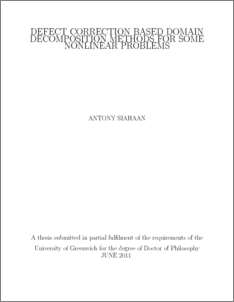# Defect correction based domain decomposition methods for some nonlinear problems

Siahaan, Antony (2011) Defect correction based domain decomposition methods for some nonlinear problems. PhD thesis, University of Greenwich.Preview
PDF
Antony_Siahaan_Defect_correction_based_domain_2011.pdf - Published Version

## Abstract

Defect correction schemes as a class of nonoverlapping domain decomposition methods offer several advantages in the ways they split a complex problem into several subdomain problems with less complexity. The schemes need a nonlinear solver to take care of the residual at the interface. The adaptive-∝ solver can converge locally in the ∞-norm, where the sufficient condition requires a relatively small local neighbourhood and the problem must have a strongly diagonal dominant Jacobian matrix with a very small condition number. Yet its advantage can be of high signicance in the computational cost where it simply needs a scalar as the approximation of Jacobian matrix. Other nonlinear solvers employed for the schemes are a Newton-GMRES method, a Newton method with a finite difference Jacobian approximation, and nonlinear conjugate gradient solvers with Fletcher-Reeves and Pollak-Ribiere searching direction formulas.

The schemes are applied to three nonlinear problems. The first problem is a heat conduction in a multichip module where there the domain is assembled from many components of
different conductivities and physical sizes. Here the implementations of the schemes satisfy the component meshing and gluing concept. A finite difference approximation of the residual of the governing equation turns out to be a better defect equation than the equality of normal derivative. Of all the nonlinear solvers implemented in the defect correction scheme, the nonlinear conjugate gradient method with Fletcher-Reeves searching direction has the best performance.

The second problem is a 2D single-phase fluid flow with heat transfer where the PHOENICS CFD code is used to run the subdomain computation. The Newton method with a finite
difference Jacobian is a reasonable interface solver in coupling these subdomain computations. The final problem is a multiphase heat and moisture transfer in a porous textile. The PHOENICS code is also used to solve the system of partial differential equations governing the multiphase process in each subdomain while the coupling of the subdomain solutions is taken care of with some FORTRAN codes by the defect correction schemes. A scheme using a modified-∝ method fails to obtain decent solutions in both single and two layers case. On the other hand, the scheme using the above Newton method produces satisfying results for both cases where it can lead an initially distant interface data into a good convergent solution. However, it is found that in general the number of nonlinear iteration of the defect correction schemes increases with the mesh refinement.

Item Type: Thesis (PhD) uk.bl.ethos.547168 decomposition method, nonlinear solvers, computer software, Q Science > QA MathematicsQ Science > QA Mathematics > QA76 Computer software School of Computing & Mathematical SciencesSchool of Computing & Mathematical Sciences > Department of Mathematical Sciences 14 Oct 2016 09:18 None None None None http://gala.gre.ac.uk/id/eprint/7144View Item